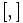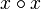# Group of nilpotency class two whose commutator map is the double of an alternating bihomomorphism giving class two

This article defines a group property: a property that can be evaluated to true/false for any given group, invariant under isomorphism
View a complete list of group properties
VIEW RELATED: Group property implications | Group property non-implications |Group metaproperty satisfactions | Group metaproperty dissatisfactions | Group property satisfactions | Group property dissatisfactions

## Definition

A group of nilpotency class two whose commutator map is the double of an alternating bihomomorphism giving class two is a group$G$ satisfying both the following:

1.$G$ is a group of nilpotency class two.
2. There is a function$\circ : G \times G \to G$ such that$(x \circ y)^2 = [x,y]$ for all$x,y \in G$, where$[ , ]$ denotes the commutator in the group,$\circ$ is a bihomomorphism,$x \circ x$ is the identity element and$x \circ y = (y \circ x)^{-1}$ for all$x,y \in G$ and$(x \circ y) \circ z$ is the identity element for all$x,y,z \in G$.

Note that for class two, the left and right conventions for commutator coincide, so it does not matter which one we pick.

This is precisely the type of group that can participate on the group side of the linear halving generalization of Baer correspondence.

## Relation with other properties

### Stronger properties

Property Meaning Proof of implication Proof of strictness (reverse implication failure) Intermediate notions
abelian group the commutator map is trivial, and its half can also be taken to be trivial CS-Baer Lie group, LCS-Baer Lie group|FULL LIST, MORE INFO
Baer Lie group CS-Baer Lie group, LCS-Baer Lie group, LUCS-Baer Lie group, UCS-Baer Lie group|FULL LIST, MORE INFO